### Home > PC > Chapter 1 > Lesson 1.1.2 > Problem1-29

1-29.
1. Simplify each expression using the rules of exponents. Homework Help ✎

1. (22) x

2. (2−5)4

3. (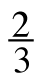) 1

4. () 2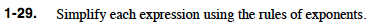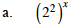22x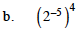2−20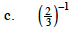What does the negative exponent mean?

$\frac{1}{\Big(\frac{2}{3}\Big)^1}$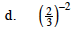This is the same as part (c), but the exponent is a 2.